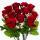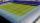# Spending

Peter spends 1/5 of his earnings on his rent, and he saves 2/7. What fraction of his earnings is left?

x =  0.5143

### Step-by-step explanation:Did you find an error or inaccuracy? Feel free to write us. Thank you!Tips to related online calculators
Need help to calculate sum, simplify or multiply fractions? Try our fraction calculator.

## Related math problems and questions:

• LeftoverAmos gets $2400 a month for his salary. He spends$1000 on rent. What fraction of his rent is leftover?
• A manA man spends 5/9 of his money on rent, and 5/16 of the remainder on electricity. If the final balance remaining is 550 find how much was spent on rentNadia spent 1/4 of her money on a shirt and 2/5 of her money on new shoes. What fraction of Nadia´s money has been spent what fraction of her money is left?
• Earning 2Rahul earns 5000 Rs, he saves 500 Rs . What's percentage of his earnings does he save? *
• DuringDuring a week, a student's spends 1/3 of her time in bed, 5/24 of her time in lesson and 1/8 of her time doing homeworks. What fraction of her time is left for doing other things?
• A sumA sum of money is shared between Peter, John and Henry in the ratio 2:3:5. a) express Henry's share as fraction of John's share. b) what fraction of the whole sum of money is John's share?
• 7 rosesPeter buys 7 roses. When he pays for it left him 4 euros. If he bought 5 roses left him 40 euros. How many euros had Peter before buying?
• Office rentFor rent 1m2 of office space is paid €165 per year. How much is the annual rent for an office as big as a football field with dimensions 71.5 m and 102.5 m?
• Equation with fractionsWhat is the simplified form of the equation fraction 4 over 5 n minus fraction 1 over 5 equals fraction 2 over 5 n? n = -2 n = 4 n = fraction 1 over 2
• A fractionI think of a fraction, if we increase its numerator and denominator by one, the value of the fraction increases by one tenth, what fraction do I think?
• Rose spendsRose spends 2 1/3 hours studying Math, 1 3/4 hours studying English, and 2 1/4 hours studying Science. Find her average time studying the three subjects.
• Sign of a expressionWhat is the sign of expression: minus 18 start fraction 9 divided by 17 end fraction plus left parenthesis minus 18 start fraction 9 divided by 17 end fraction right parenthesis?
• Farmer PeterFarmer Peter paints 12 chicken coop. He started painting this day morning. Now he only has 1/4 of the chicken coop left to paint this afternoon. How many chicken coops did farmer Peter paint this morning?
• MoneyPeter has 2x more money than Michael. But if Peter gave Michael € 16 Michael would have 3.5 times more money than Peter. Determine how much money was originally Peter and how much Michael.
• WithdrawalIf I withdrew 2/5 of my total savings and spent 7/10 of that amount. What fraction do I have in left in my savings?
• MichaelMichael had a bar of chocolate. He ate 1/2 of it and gave away 1/3. What fraction had he left?
• Over fractionSolve equation: p over 5 equals fraction numerator 7 p over denominator 6 end fraction plus 5